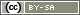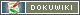# valueMean()

`float valueMean(string question)`

`float valueMean(string question, string|array items)`

`float valueMean(array variables)`

Calculates the mean (average, expectation value) of the answer codes for all items in a question (e.g. a scale), or for a list of variables.

• question – ID of a question (as a string)
• items – A list (string or array) of items
• variables – A list (array) of variable IDs as stated in the Variables Overview

Note This works in the same way as `valueSum()` – the parameters are described in this chapter in detail with various examples.

## Example filter

In the following example, question “AB02” will be displayed if the mean in the scale “AB01” is at least 1.5.

```\$mean = valueMean('AB01');
if (\$mean >= 1.5) {
question('AB02');
}```

## Example items

The following PHP code calculates the mean of the items 2, 4, 6, 8 and 10 in question AB02.

`valueMean('AB01', [2, 4, 6, 8, 10])`

## Example variables

The following PHP code calculates the mean of the variables AB03_01, AB03_02, BB01_02 and BB01_04.

`valueMean(['AB03_01', 'AB03_02', 'BB01_02', 'BB01_04'])`

Whether a Array is defined in one line or over several lines is irrelevant for the function, but can improve clarity.

```valueMean([
'AB03_01', 'AB03_02',
'BB01_02', 'BB01_04'
])```

## Example weighting

It is not possible to calculate a weighted average using “valueMean()”, but using a FOR loop and simple arithmetic operations such a weighted average can be easily calculated using PHP.

```\$weights = [
'AB03_01' => 1.1,
'AB03_02' => 1.4,
'BB01_02' => 0.7,
'BB01_04' => 0.8
];
\$sumValue = 0;
\$sumWeight = 0;

foreach (\$weights as \$varID => \$weight) {
\$val = (float)value(\$varID);
// Exclude missing data (≤0)
if (\$val > 0) {
\$sumValue+= \$val * \$weight;
\$sumWeight+= \$weight;
}
}

// Mean = sum divided by count
if (\$sumWeight == 0) {
\$mean = -1;  // No data
} else {
\$mean = \$sumValue / \$sumWeight;
}```